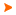Podaci o VU Početna stranicaArhiva nastavnih programaArhiva ak. kalendaraEconometrics
 Kratica: Opterećenje: 30(P) + 0(S) + 0(SJ) + 30(A) + 0(TJ) + 0(PK) + 0(T)
 Nositelji: izv. prof. dr. sc. Tunjo Perić Izvođači: izv. prof. dr. sc. Josip Arnerić ( Auditorne vježbe, Predavanja ) Opis predmeta: COURSE OBJECTIVES Specification, estimation and evaluation of goodness of fit of econometric model. INTRODUCTION Concept of econometrics - possibilities of applications. Linear and nonlinear econometric models. Observed data structure. Data transformation. CROSS-SECTIONAL LINEAR ECONOMETRIC MODEL Linearity of econometric model. Estimating criteria. Ordinary least squares method (OLS). Maximum likelihood estimation method (MLE). Assumptions of econometric model and diagnostic checking. Reliability testing of an estimated econometric model and, particulary, individual and joint significance test of estimated parameters. Wald test. Redudant and omitted variable test. LR test. Autocorrelation between the random disturbances, heteroscedasticity, generalized least squres method (GLS). White test. LM test. Newey-West standard errors. Perfect multicollinearity and "near" milticollinearity, ridge regression. Dummy variables inclusion in econometric models as independent variables. Interaction terms with dummy variables. Applications with econometric software - EViews. TIME SERIES ANALYSIS Univariate time series analysis. Time series decomposition (additive and multiplicative). Seasonal adjustment (Census I method). Seasonal dummies. Linear models of stationary time series. AR models, MA models, ARMA models. ACF and PACF. Integrated time series. Testing of white noise process. Unit root tests. DF and ADF test. Forecasting using ARIMA(p,d,q) model. Model selection according to information criteria (AIC, SIC, HQIC). Box-Jenkins approach. Dynamic models. ADL(1,1) model and PA model. Error correction model EC. Engle-Granger approach for testing of cointegration. Impulse response function IRF. Testing for endogeneity. Granger causality test. Multivariate time series analysis. VAR models, SVAR models. VECM representation of VA(p) model. Cointegration vector and spped of adjustment. IRF and OIRF. Cholesky factorization of covariance matrix. Testing for cointegration in VAR(p) model. VECM and variance decomposition. Applications with econometric software - EViews.
 Jezici na kojima se održava nastava: - - -
 Obavezna literatura: 1. Maddala,G.S.(2001), Introduction to Econometrics, Third Edt., John Wiley&Sons, Ltd.
 Preporučena literatura: 2. Dougherty,C.: Introduction to Econometrics, Oxford University Press, 2002 Green, W. H.: Econometric Analysis, Seventh Edt., Prentice Hall, 2012 Gujarati, D.N.: Basic Econometrics, Third Edt., McGraw-Hill, 1995 Heij at al.: Econometric Methods with Applications in Business and Economics, Oxford University Press, 2004 Wooldridge, J. M.: Introductory Econometrics- A Modern Approach, Second Edt., South western, 2002 Maddala,G. S.: Introduction to Econometrics, Third Edt., John Wiley & Sons, 2001Legenda P - Predavanja S - Seminar SJ - Vježbe iz stranog jezika A - Auditorne vježbe TJ - Vježbe tjelesnog odgoja PK - Vježbe u praktikumu T - Terenske vježbe * - Predmet se ne polaže| Početna stranica | Visoka učilišta | Korisničke stranice | | Na vrh | Srce - Sveučilišni računski centar Sveučilišta u Zagrebu